``````library(Lahman)
library(ggplot2)
library(dplyr)
library(ggalt)
library(ggExtra)``````
``## Warning: package 'ggExtra' was built under R version 3.4.3``
``library(ggcorrplot)``
``## Warning: package 'ggcorrplot' was built under R version 3.4.3``
``````Pitch14 <- Pitching %>% filter(yearID == "2014")
Pitch13 <- Pitching %>% filter(yearID == "2013")
Pitch12 <- Pitching %>% filter(yearID == "2012")
Pitch11 <- Pitching %>% filter(yearID == "2011")
Pitch10 <- Pitching %>% filter(yearID == "2010")``````
``````scatterplot <- ggplot(data = Pitch14, aes(x = IPouts, y = ERA)) +
geom_point(aes(col = W, size = ER)) +
geom_smooth(method = "loess", se = FALSE) +
xlim(c(0, 750)) +
ylim(c(0, 15)) +
labs(title = "MLB ERA, Wins, and Earned Runs", subtitle = "from Lahman data set",
x = "Outs (Innings Pitched)", y = "Earned Run Average", caption = "for 2014 season")
scatterplot``````
``## Warning: Removed 27 rows containing non-finite values (stat_smooth).``
``## Warning: Removed 27 rows containing missing values (geom_point).``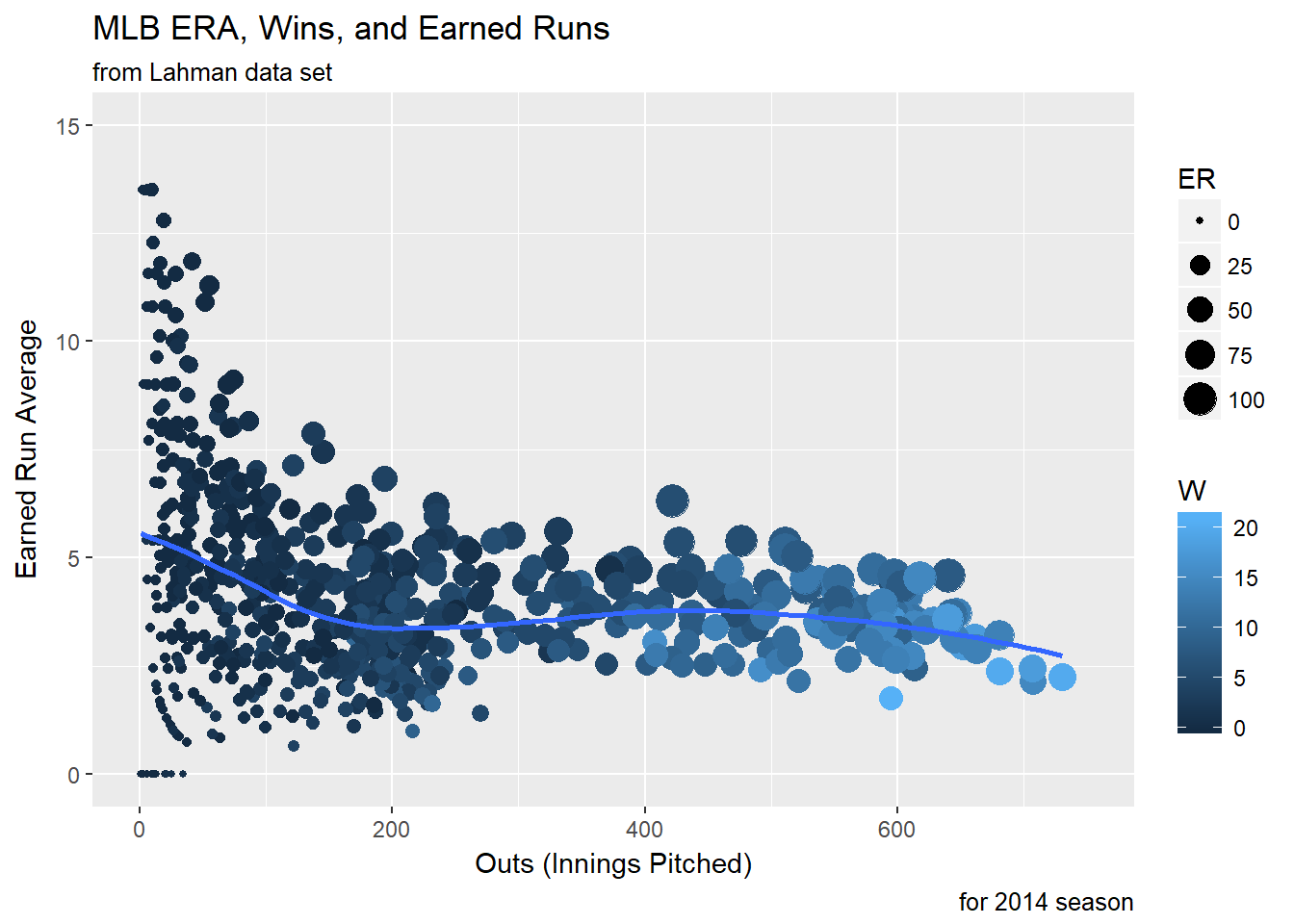``ERA_select <- Pitch14[Pitch14\$IPouts > 650 & Pitch14\$ERA < 5,]``
``````scatterplot_enc <- ggplot(data = Pitch14, aes(x = IPouts, y = ERA)) +
geom_point(aes(col = W, size = ER)) +
geom_smooth(method = "loess", se = FALSE) +
xlim(c(0, 750)) +
ylim(c(0, 15)) +
geom_encircle(aes(x = IPouts, y = ERA), data = ERA_select, color = "red", size = 2, expand = 0.08) +
labs(title = "MLB ERA, Wins, and Earned Runs", subtitle = "from Lahman data set",
x = "Outs (Innings Pitched)", y = "Earned Run Average", caption = "for 2014 season")
scatterplot_enc``````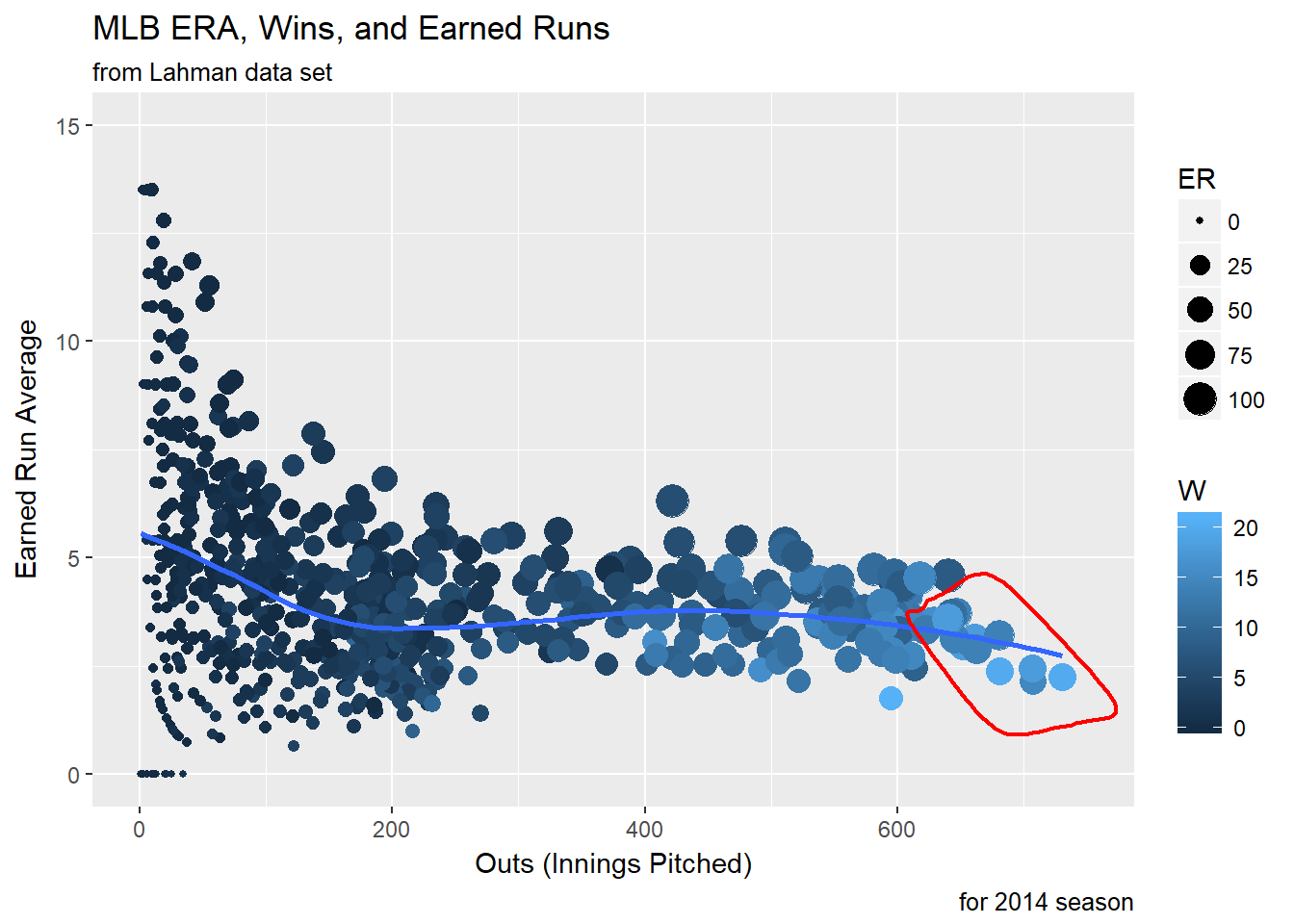``theme_set(theme_bw())``
``````ggplot(data = Pitch14, aes(x = IPouts, y = ERA)) + geom_point() +
geom_smooth(method = loess, se = FALSE) +
xlim(c(0, 750)) +
ylim(c(0, 15)) +
labs(title = "MLB ERA by Innings Pitched", subtitle = "from Lahman data set",
x = "Outs (Innings Pitched)", y = "Earned Run Average", caption = "for 2014 season")``````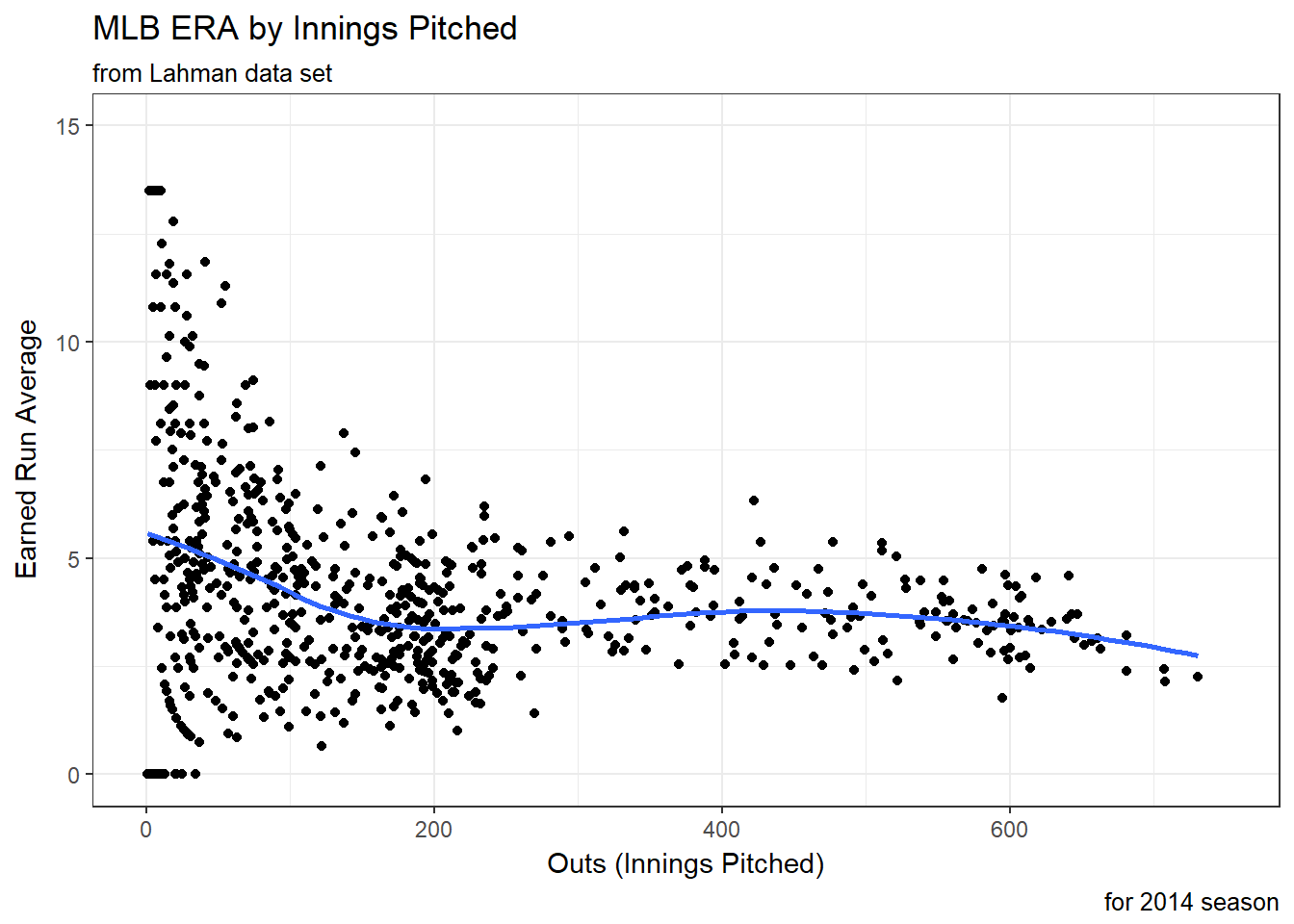``dim(Pitch14)``
``##  746  30``
``````ggplot(data = Pitch14, aes(x = IPouts, y = ERA)) + geom_jitter(width = 0.5, size = 1) +
geom_smooth(method = loess, se = FALSE) +
xlim(c(0, 750)) +
ylim(c(0, 15)) +
labs(title = "MLB ERA by Innings Pitched", subtitle = "from Lahman data set",
x = "Outs (Innings Pitched)", y = "Earned Run Average", caption = "for 2014 season")``````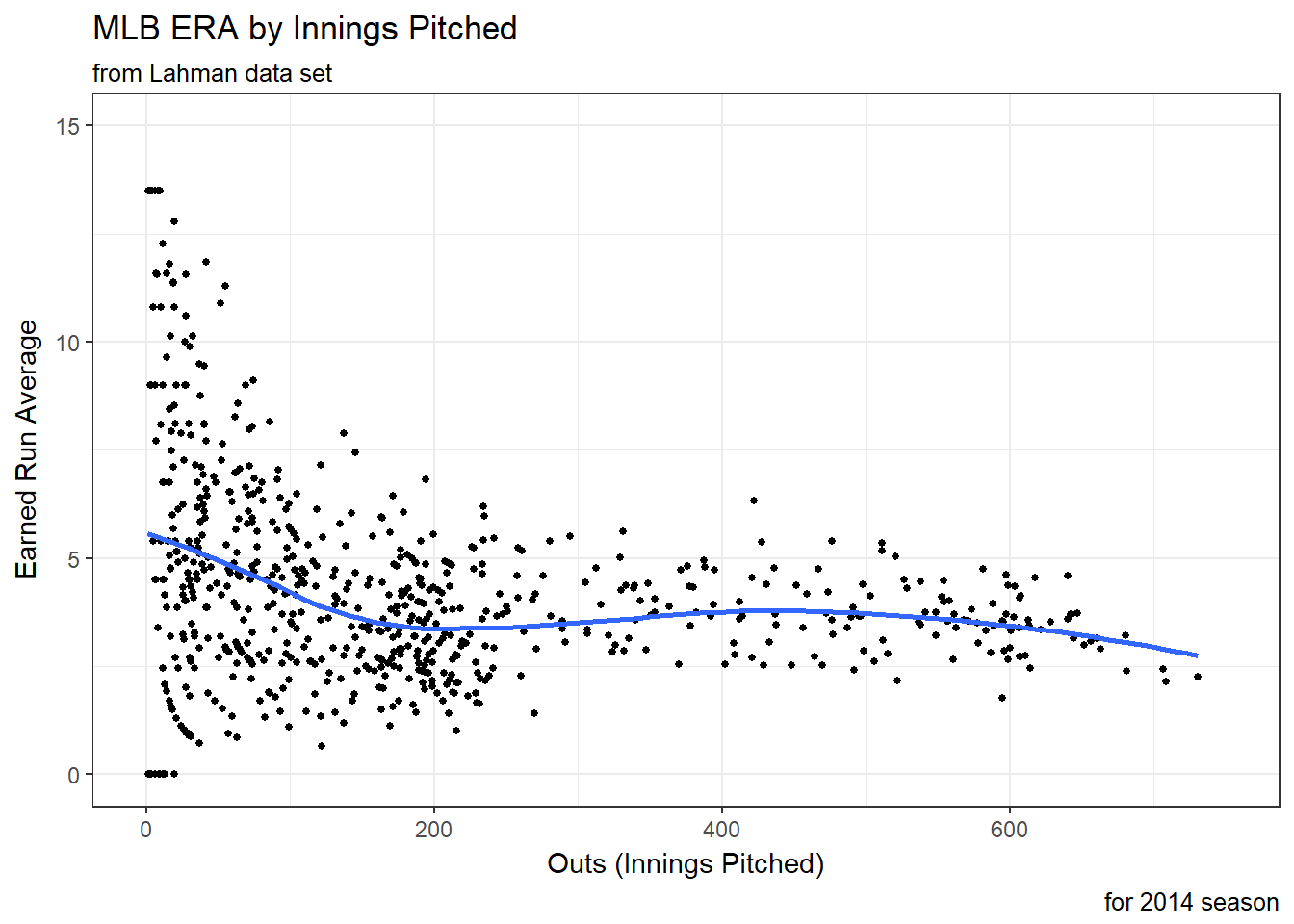``````ggplot(data = Pitch14, aes(x = IPouts, y = ERA)) + geom_count(aes(size = HR), col = "firebrick") +
xlim(c(0, 750)) +
ylim(c(0, 15)) +
labs(title = "MLB ERA by Innings Pitched and Home Runs", subtitle = "from Lahman data set",
x = "Outs (Innings Pitched)", y = "Earned Run Average", caption = "for 2014 season")``````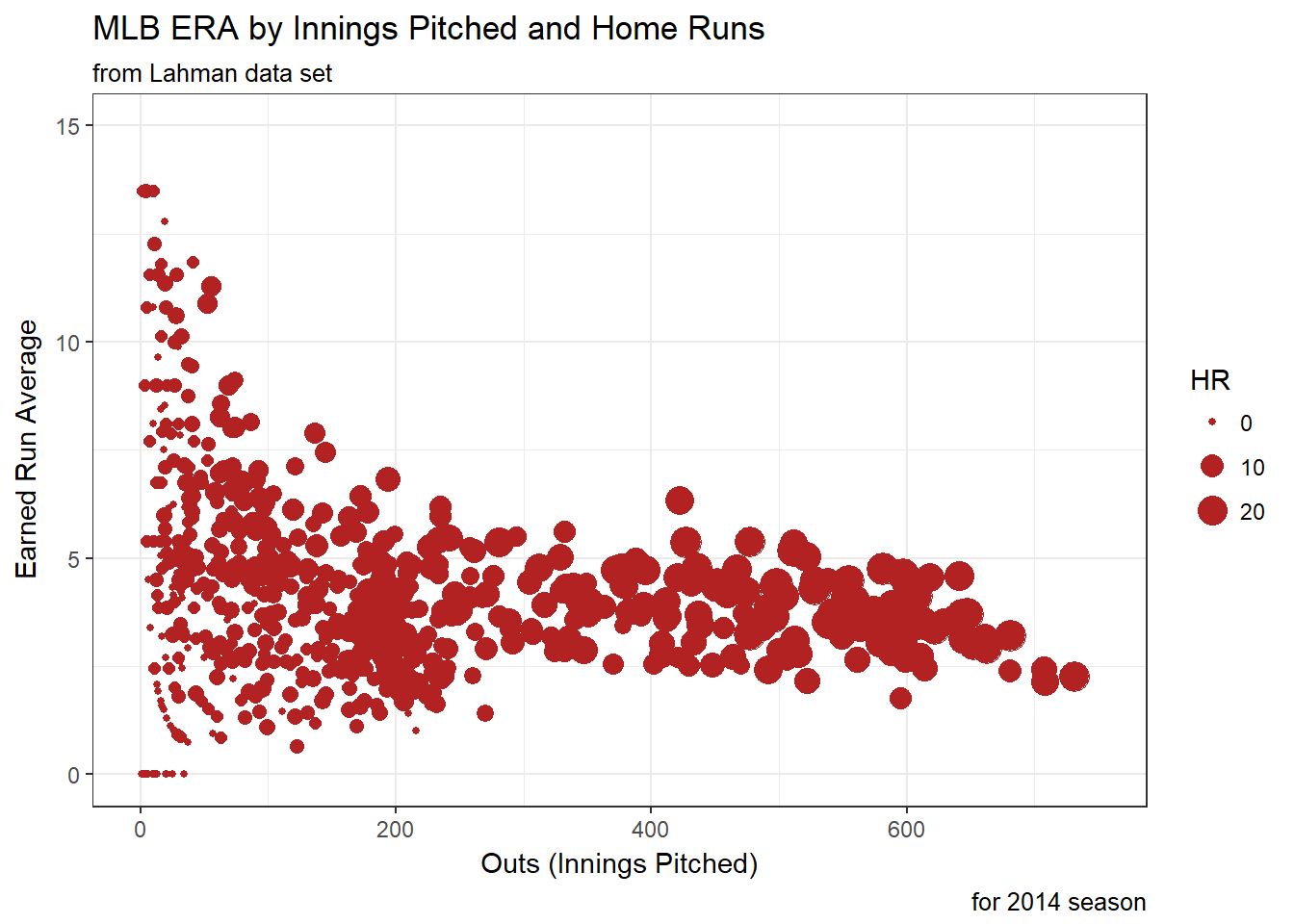``ERA14 <- Pitch14 %>% group_by(teamID) %>% summarize(era = sum(ER)/sum(IPouts)*27, na.rm = TRUE) %>% arrange(era)``
``View(ERA14)``
``BestTeamERA <- Pitch14[Pitch14\$teamID %in% c("WAS", "SEA", "OAK", "SDN", "ATL"), ]``
``````ggplot(BestTeamERA, aes(x = IPouts, y = ERA)) + geom_smooth(aes(col = teamID), method = loess, se = FALSE) +
geom_jitter(aes(col = teamID, size = HR)) +
xlim(c(0, 750)) +
ylim(c(0, 15)) +
labs(title = "Five Best Team ERAs in 2014", subtitle = "from Lahman data set",
x = "Outs (Innings Pitched)", y = "Earned Run Average", caption = "for 2014 season")``````